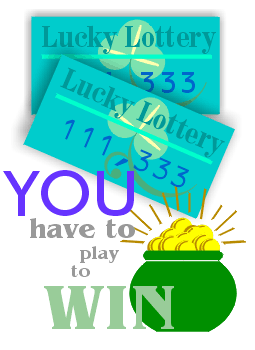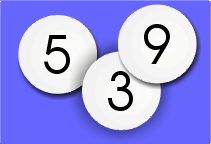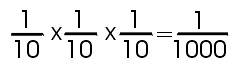Wouldn't it be great to win the lottery?All you have to do is buy a ticket. But what is the likelihood that your ticket will be drawn? Probability will tell you.

 The probability of winning a lottery game depends on the type of game. In a 3-digit lottery game, where the object is to pick the correct number from 000 to 999, your chances of winning are 1:1000.Most games like this have numbered balls from 0 to 9. There are 3 sets of balls from which the numbers are drawn.The probability for one specific ball being selected is 1:10. Because there are 3 numbers to be chosen, 3 different selections from the10 balls must be made. The probability of any 3-digit number being selected is the product of the 3 ratios.By boxing a number (choosing any combination of the 3 digits)
the probability of winning increases.

Why? Because boxing a number gives you more combinations, therefore, more opportunities to win.

A number like 232 can create these combinations:
 232 223 322
How many 3-digit combinations can be made from the numbers
4, 5 and
6?